# Lesson 4: Unidirectional pure loads

In this lesson, we will delve into the unidirectional pure loads and their corresponding stress and deformation states.

1. Hooke’s Law and Unidirectional Deformation
• In unidirectional deformation, only elongation or compression occurs.
• Deformation State: Stress and deformations are not always present in all directions; some tensor components can be zero.
• Stress and Deformation State: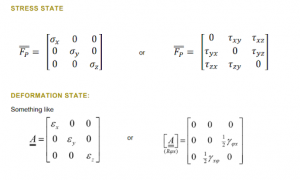• Hooke’s Law: Stress required for deformation scales linearly with respect to that deformation.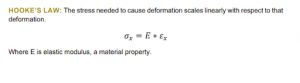1. Central Tension and Compression
• Force acts at the centroid of the cross-section and parallel to it.
• Stress State: Stress can be calculated by dividing the normal force by the cross-sectional area.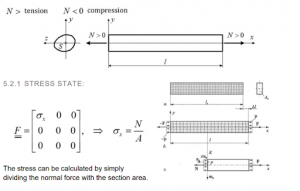• Hooke’s Law and deformation:
• Longitudinal Deformation:
• Transversal Deformation: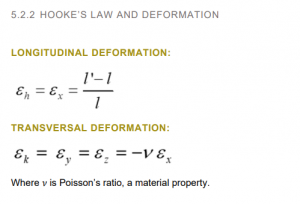1. Pure Shear
• Force acts perpendicular to the cross-section in a single line without bending moment.
• In reality, pure shear is often paired with bending, but simplifications can be made in specific cases.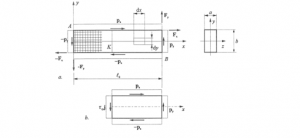• Stress State and Hooke’s Law and Shear deformation: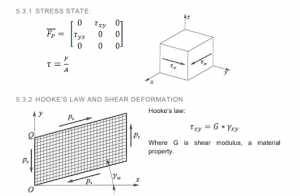1. Pure Twist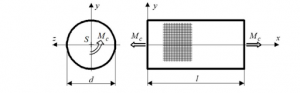• A moment vector acts at the centroid of the cross-section and parallel to it.
• Stress State: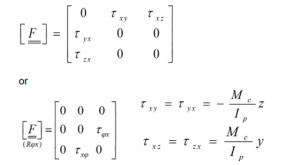• Stress Distribution: Stress exists only where there is material.
• Twist Deformation:
• Defomation State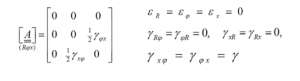1. Pure, Straight Bending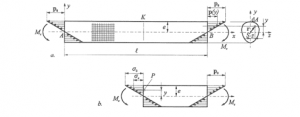• A single moment bends the entire beam.
• Stress State: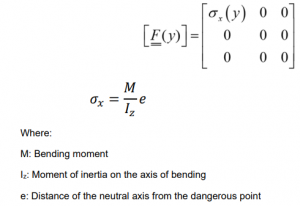• Stress Distribution: The most critical point is the one farthest from the neutral axis, where bending stress is maximum.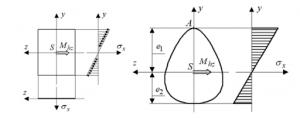• Dangerous Point:The point on the cross-section which is farthest from the neutral axis. The stress from bending is maximum at this point.
• According to Euler-Bernoulli beam theory, each cross-section of the beam is planar and oriented at 90 degrees to the neutral axis.
• Deformation State: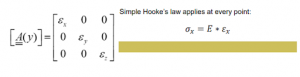Understanding these unidirectional pure loads and their corresponding stress and deformation states is crucial for analyzing and designing structures and components subjected to these types of loads. It forms the foundation for more complex structural analyses and real-world engineering applications.